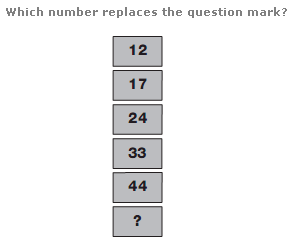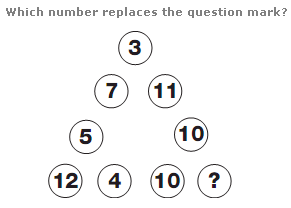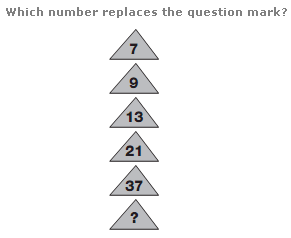# Puzzles - Number puzzles

### Exercise :: Number puzzlesAnswer : 57 Explanation : As you work down the column, numbers follow the sequence of Square Numbers from 16 to 81, subtracting 4, then 8, then 12 etc.Answer : 1 Explanation : Splitting the diagram into 3 smaller triangles, with 3 circles each, the sum of the numbers in each smaller triangle is always 21.Answer : 69 Explanation : Starting at the top and working down, double each number and subtract 5 to give the next number down.